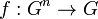Reducible multiary group

Definition

Recall that a multiary group is a$n$-ary group for some$n \ge 2$. A$n$-ary group with multiplication$f:G^n \to G$ is termed reducible if it satisfies the following equivalent conditions:

No. Shorthand Statement with symbols Note
1 arises from a group there exists a group structure on$G$ such that$f(a_1,a_2,\dots,a_n) = a_1a_2\dots a_n$ for all (possibly repeated)$a_1,a_2,\dot,a_n \in G$, with the multiplication on the right being as per the group structure. For more on this, see group is n-ary group for all n If we're assuming this, we don't need to check the$n$-ary associativity and unique solution conditions; they follow automatically.
2 existence of neutral element there exists a neutral element$e \in G$ for$f$:$f$ evaluated at any tuple where$n - 1$ of the entries are equal to$e$ and the remaining entry is$a \in G$, gives output$a$. This is true regardless of where we place$a$ and is also true if$a = e$. Note that we do need to separately check the associativity and unique solution conditions.

Equivalence of definitions

Further information: equivalence of definitions of reducible multiary group